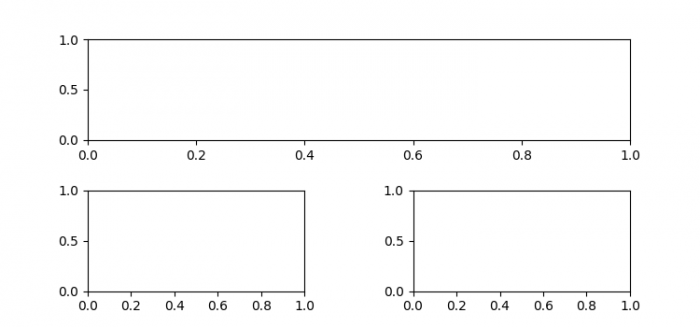# How to increase the spacing between subplots in Matplotlib with subplot2grid?

To increase the spacing between subplots with subplot2grid, we can take the following steps −

• Set the figure size and adjust the padding between and around the subplots.

• Add a grid layout to place subplots within a figure.

• Update the subplot parameters of the grid.

• Add a subplot to the current figure.

• To display the figure, use show() method.

## Example

from matplotlib import pyplot as plt

plt.rcParams["figure.figsize"] = [7.50, 3.50]
plt.rcParams["figure.autolayout"] = True

ax = plt.GridSpec(2, 2)
ax.update(wspace=0.5, hspace=0.5)

ax1 = plt.subplot(ax[0, :])
ax2 = plt.subplot(ax[1, 0])
ax3 = plt.subplot(ax[1, 1])

plt.show()

## Output